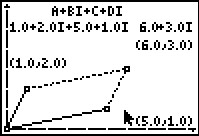# Activities

••• ##### Subject Area

• Math: Precalculus: Complex Numbers

• ##### Author9-12

20 Minutes

• ##### Device
• TI-83 Plus Family
• TI-84 Plus
• TI-84 Plus Silver Edition
•TI-84 Plus C Silver Edition
•TI-84 Plus CE
• ##### Software

TI Connect™
TI Connect™ CE

## It's a Parallelogram, You Say?#### Activity Overview

Students represent complex numbers in the complex plane as points or vectors and display the sum and difference of two complex numbers as diagonals of the parallelograms they define.

#### Key Steps

•In this introductory activity, students will explore the addition and subtraction of complex numbers in the complex coordinate plane using vector addition with the Cabri™ Jr. Application. They are first presented with how to graph a complex number on a coordinate plane.

•Then students explore of the concept of vector addition. They are to determine the relationship between the resultant of the parallelogram and the values of the complex numbers being added.

Students are then given addition and subtraction problems to solve.